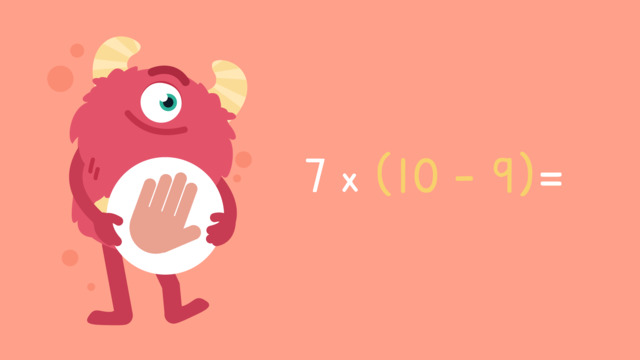# Operations: Parenthesis — Let's Practice!Rating

Be the first to give a rating!
The authorsTeam Digital
Operations: Parenthesis — Let's Practice!
CCSS.MATH.CONTENT.3.OA.B.5

## Basics on the topicOperations: Parenthesis — Let's Practice!

Today we are practicing operations: parenthesis with Razzi! This video contains examples to help you further practice and grow confident in this topic.

### TranscriptOperations: Parenthesis — Let's Practice!

Razzi says get these items ready, because today we're going to practice operations: Parenthesis. It's time to begin! Remember, always solve inside the parentheses first! Solve seven minus three times two. Pause the video to work on the problem, and press play when you are ready to see the solution! Solve inside the parentheses first. Seven minus three equals four. Rewrite the equation. Solve four times two, which is eight. Did you also get eight? Let's tackle the next problem! Solve sixty-eight minus four times ten. Pause the video to work on the problem, and press play when you are ready to see the solution! Solve inside the parentheses first. Four times ten equals forty. Rewrite the equation. Solve sixty-eight minus forty, which is twenty-eight. Did you also get twenty-eight? Let's tackle the final problem! Solve five plus two times ten minus nine. Pause the video to work on the problem, and press play when you are ready to see the solution! Solve inside the left parentheses first. Five plus two equals seven. Rewrite the equation. Solve inside the remaining parentheses. Ten minus nine is one. Rewrite the equation. Solve seven times one, which is seven. Did you also get seven? Razzi had so much fun practicing with you today! See you next time!

## Operations: Parenthesis — Let's Practice! exercise

Would you like to apply the knowledge you’ve learned? You can review and practice it with the tasks for the video Operations: Parenthesis — Let's Practice!.
• ### Select the action to do first.

Hints

Remember, in a problem like (12 - 4) x 2, we would rewrite the problem as 8 x 2.

Solution

When we are solving a problem with parentheses, always solve the problem inside the parenthesis first.

For example:

(30 - 15) - 10 = __

15 - 10 = 5

• ### How can we rewrite these problems?

Hints

Remember, to rewrite the problems, you must solve inside the parenthesis first.

Look at this example:

(45 - 20) + (10 - 5) can be rewritten as 25 + 5.

(45 - 20) gives us 25, (10 - 5) gives us 5.

Solution

(68 - 8) x 1 can be rewritten as: 60 x 1.

• (68 - 8) is 60, so the problem becomes 60 x 1.
(17 + 10) - (10 + 10) can be rewritten as: 27 - 20.
• (17 + 10) is 27, and (10 + 10) is 20, so the problem becomes 27 - 20.
55 + (20 - 5) can be rewritten as: 55 + 15.
• (20 - 5) is 15, so the problem becomes 55 + 15.

• ### What is (34 - 10) + (14 - 7)?

Hints

Remember, to rewrite the problem, solve what is inside the parenthesis first.

Look at this example:

(50 - 5) - (20 x 2) can be rewritten as 45 - 40.

45 - 40 = 5.

Solution

(34 - 10) is 24, and (14 - 7) is 7, so we can rewrite the problem as: 24 + 7.

24 + 7 = 31

• ### Solve the equations.

Hints

Always solve inside the parenthesis first. Rewrite the problem on paper as you solve step by step.

Think about what to do first: (8 + 12) x 2.

First, solve inside the parenthesis: (8 + 12) = 20.

Then, solve the problem!

Solution

(8 + 12) x 2 = 40.

(8 + 12) is 20, so we rewrite the equation as: 20 x 2.

20 x 2 = 40

8 - (35 - 33) = 6.

(35 - 33) is 2, so we rewrite the equation as: 8 - 2.

8 - 2 = 6.

6 + (6 x 2) = 18.

(6 x 2) is 12, so we rewrite the problem as: 6 + 12.

6 + 12 = 18.

• ### Choose the correct answer.

Hints

Remember, solve the equation in the parenthesis first: (9 - 4) = ?

After you get the answer for 9 - 4 add 11 to it.

Solution

(9 - 4) + 11 = 16

(9 - 4) = 5, so we rewrite the equation as: 5 + 11.

5 + 11 = 16.

• ### Solve the equations.

Hints

Remember, rewrite the problem by solving inside the parenthesis first.

Look at this example: (4 + 8) x (10 - 9) = ?

We solve inside the parenthesis first. (4 + 8) = 12, and (10 - 9) = 1, so we rewrite the problem as: 12 x 1.

12 x 1 = 12

Solution

(24 + 8) - (4 + 1) = 27. (24 + 8) is 32, and (4 + 1) is 5, so we rewrite it as: 32 - 5 = 27.

56 - (60 - 10) = 6. (60 - 10) is 50, so we rewrite it as: 56 - 50 = 6.

(75 - 15) + (40 - 30) = 70. (75 - 15) is 60, and (40 - 30) is 10, so we rewrite it as: 60 + 10 = 70.

(4 x 2) + (6 x 2) = 20. (4 x 2) is 8, and (6 x 2) is 12, so we rewrite is as: 8 + 12 = 20.